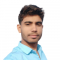## Positive and Negative Logic in Digital ElectronicsUpdated on 26-Dec-2022 18:27:33

7K+ Views

In digital electronics, a logic or logic function is one that follows the rules that governs a logical statement. Logics are implemented by using switching networks, where a switching network is one that is designed by interconnecting a finite number of switches. Example − Let "𝒚" is a logic function, then it may be expressed as, $$\mathrm{Y = f\left ( x_{i} \right ) }$$ Where, xi represents the inputs to the logic network or switching network, and y is the output of the network. There is another term associated with the logic function that is the truth table. The truth ... Read More